6.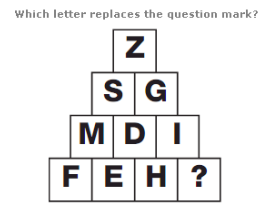A. T B. R C. G D. F

Explanation: :

The numerical values of the letters in each row add up to 26 each time.

7.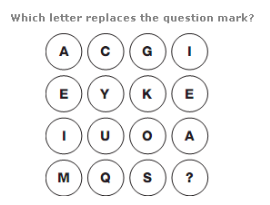A. W B. E C. R D. T

Explanation: :

Starting at the top left and moving down, then right one column and up etc in a snakes and ladders pattern, letters move through the alphabet 4 letters at a time.

8.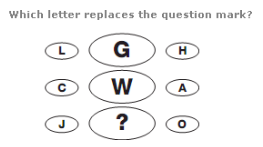A. B B. N C. M D. K

Explanation: :

In each row, add the numerical value of the left and right hand letters together and write the letter with the reverse alphabetical value in the centre.

9.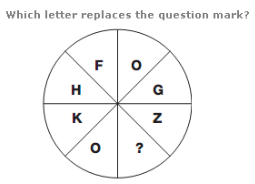A. L B. A C. S D. T

Explanation: :

Starting at F and moving anti-clockwise, letters move through the alphabet in steps of 2, 3, 4, 5 etc.

10.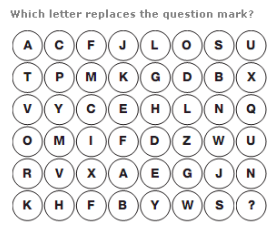A. D B. O C. P D. E